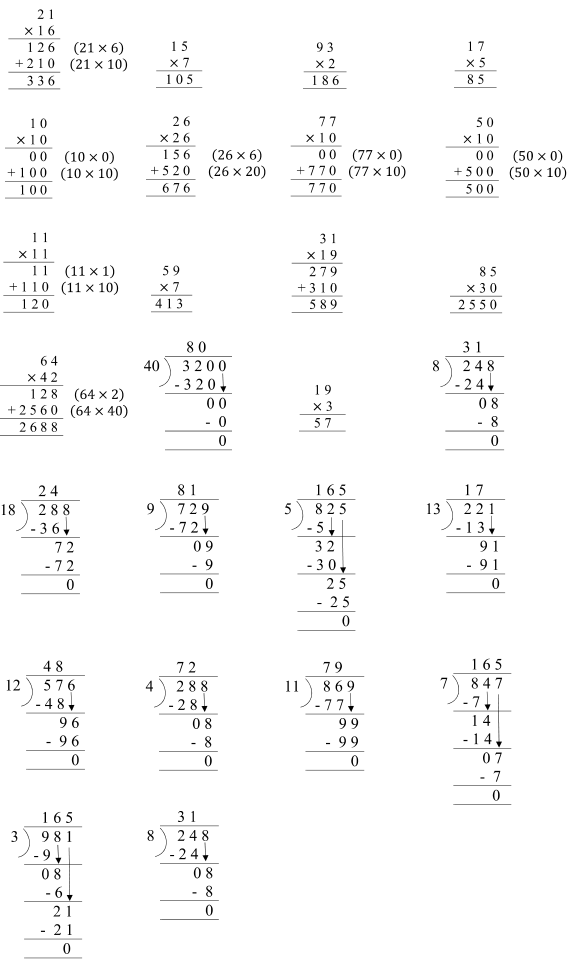# NCERT Solutions for Chapter 13 Ways To Multiply And Divide Class 5 MathsWays To Multiply And Divide Questions and Answers

 Chapter Name Ways To Multiply And Divide NCERT Solutions Class CBSE Class 5 Textbook Name Maths Related Readings NCERT Solutions for Class 5NCERT Solutions for Class 5 Maths Summary of Ways To Multiply And Divide

Page No 171:

Question 1:

Use Bela's method to multiply these numbers.

(a) 32 × 46

(b) 67 x 18

Do these in your notebook using Bela's method. (a) 47 x 19 (b) 188 × 91 (c) 63 × 57 (d) 225 × 22 (e) 360 x 12 (f) 163 × 42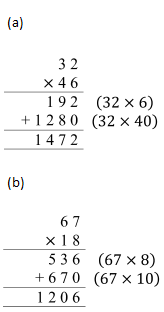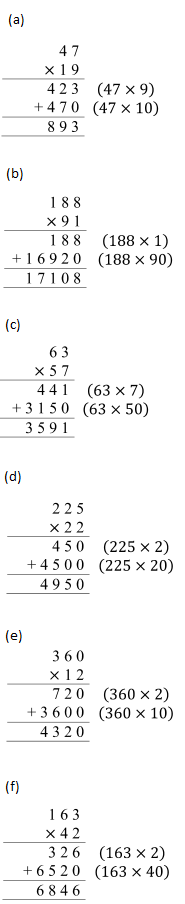Page No 172:

Question 1:

• Shantaram is a special cook who comes only on party days. Last year he was called for only 28 days. For each day he has to be paid Rs 165. Find out how much money he will get in all.
• If he is called for all days of the year, how much salary will he get?

165×365        _ _ _                  165×5              _ _ _

165×60+49500                       165×300

• Now find the salaries of the minister and horse rider for 1 year.

• Salary paid to Shantaram for 1 day = Rs 165 Salary paid to Shantaram for 28 days = Rs 165 × 28 = Rs 4,620

Working:

165
× 28
1320    (165 x 8)
+3300    (165 x 20)
4620

Thus, Shantaram will be paid Rs 4,620 for 28 days.

We know 1 year = 365 days

∴ Salary to be paid to Shantaram for 365 days = 365 × Rs 165 = Rs 60,225

Working:

165
×365
825   (165 × 5)
9900   (165 x 60)
+49500  (165 x 300)
60225

Thus, Shantaram will be paid Rs 60,225 for a year.

• Salary paid to the minister for 1 day = Rs 195 Salary paid to the minister for 365 days = Rs 195 x 365 = Rs 71,175

Working:

195
×365
975  (195 x5)
11700  (195 x 60)
+58500  (195 x 300)
71175

Thus, the minister is paid Rs 71,175 for a year.

Salary paid to the horse rider for 1 day = Rs 95 Salary paid to the horse rider for 365 days = 365 x Rs 95 = Rs 34,675

Working:

365
× 95
1825   (365 x 5)
+32850   (365 x 90)
34675

Thus, the horse rider is paid Rs 34,675 for a year.

Question 2: Years and Years

(a) Sohan drinks 8 glasses of water every day.

• How many glasses will he drink in one month? ____
• How many glasses will he drink in one year?
• If 125 people living in a colony drink 8 glasses of water in a day, how much water will they drink in a year?

(b) If Soha's heart beats 72 times in one minute, how many times does it beat in one hour?

• Now find out how many times it beats in one day.
• Count your own heart beats to find out how many times your heart beats in one week.

(c) A baby elephant drinks around 12 L of milk every day. How much milk will it drink in two years?

(d) A baby blue whale drinks around 200 L of milk in one day. Just imagine how much milk that is! Find out in how many days your family would use 200 L milk. How much milk would the baby blue whale drink in eight months?

(a) Number of glasses of water consumed by Sohan in 1 day = 8 We know 1 month 30 days

∴ Number of glasses of water consumed by Sohan in 30 days = 30 × 8 = 240

Working:
30
x 8
240

Thus, Sohan will drink 240 glasses of water in one month.

• We know 1 year = 365 days. ∴Number of glasses of water consumed by Sohan in 365 days = 365 x 8 = 2,920

Working:

365
× 8
2920

Thus, Sohan will drink 2,920 glasses of water in one year.

• Number of glasses of water consumed by 125 people of the colony in 1 day = 8

∴ Number of glasses of water consumed by 125 people of the colony in 365 days = 365 x 8 = 2,920

Working:

365
× 8
2920

Thus, 125 people of the colony will drink 2,920 glasses of water in one year.

(b) Number of times Soha's heart beats in 1 minute = 72

We know, 1 hour = 60 minutes

∴ Number of times Soha's heart beats in 60 minutes = 72 × 60 = 4,320

Working:

72
× 60
00   (72 × 0)
+4320   (72 x 60)
4320

Thus, Soha's heart beats 4,320 times in one hour.

• We know 1 day = 24 hours 1 hour = 60 minutes

∴ 24 hours = 24 × 60 minutes = 1,440 minutes Number of times Soha's heart beats in 1 minute = 72

∴ Number of times Soha's heart beats in 1,440 minutes = 1440 × 72 = 1,03,680

Working:

1440
× 72
2880  (1440 x 2)
+100800  (1440 x 70)
103680

Thus, Soha's heart beats 1,03,680 times in one day.

My heart beats 72 times in one minute. We know 1 week = 7 days 1 day = 1,440 minutes 7 days = 7 x 1440 minutes = 10,080 minutes

Working:

1440
x 7
10080

∴ Number of times my heart beats in 10,080 minutes = 10080 × 72= 7,25,760

Working:

10080
× 72
20160   (10080 x 2)
+705600   (10080 x 70)
725760

Thus, my heart beats 7,25,760 times in one week.

(c) Amount of milk consumed by the baby elephant in 1 day = 12 L We know 1 year = 365 days 2 years = 2 × 365 days = 730 days

Working:

365
x 2
730

Now, Amount of milk consumed by the baby elephant in 730 days = 730 ×12 L = 8,760 L

Working:

73
× 12
1460   (730 x 2)
+7300   (730 x 10)
8760

Thus, the baby elephant will drink 8,760 L of milk in two years.

(d) Amount of milk consumed by the baby blue whale in 1 day = 200 L My family uses 5 L milk every day.

∴ Number of days in which 200 L of milk is consumed by my family = 200 ÷ 5 = 40

Working: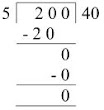We know I month = 30 days. ∴ Amount of milk consumed by the baby blue whale in 30 days = 200 × 30 = 6,000 L

Working:

200
×30
000    (200 x 0)
+6000   (200 × 30)
6000

Thus, Amount of milk consumed by the baby blue whale in 1 month 6,000 L Amount of milk that will be consumed by the baby blue whale in 8 months = 6000 L x 8 = 48,000 L

Working:

6000
x 8
48000

Thus, the baby blue whale will drink 48,000 L of milk in eight months.

Page No 173:

Question 1:

Karunya bought three fields.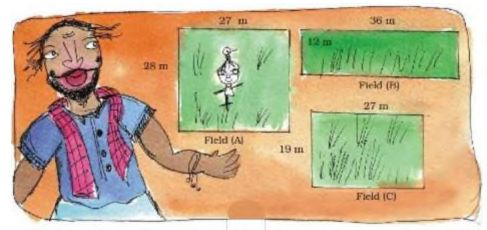Page No 173:

Find the area of all the three fields. Field

(A) ____ square metre. Field

(B) ____ square metre. Field

(C) ____ square metre. He bought field

(A) at the rate of Rs 95 for a square metre, field

(B) at Rs 110 for a square metre and field

(C) at Rs 120 for a square metre.

Find the cost of all three fields.

Length of field A = 27 m

Breadth of field A = 28 m Area of field A = Length x Breadth = 27 × 28 = 756 square metres

Working:

28
× 27
196  (28 × 7)
+560  (28 x 20)
756

Length of field B = 36 m Breadth of field B = 12 m Area of field B = Length x Breadth = 36 x 12 = 432 square metres

Working:

36
X 12
72  (36 × 2)
+360  (36 x 10)
432

Length of field C = 27 m Breadth of field C = 19 m Area of field C = Length x breadth = 27 x 19 = 513 square metres

Working:

27
× 19
243   (27 x 9)
+270   (27×10)
513

• Cost of 1 square metre of field A = Rs 95

Now, Area of field A = 756 square metres

∴ Cost of 756 square metres of field A = 756 × Rs 95 = Rs 71,820

Working:

756
×95
3780   (756 x 5)
+68040   (756 × 90)
71820

Cost of I square metre of field B = Rs 110

Now, Area of field B = 432 square metres

∴ Cost of 432 square metres of field B = Rs 432 x 110 = Rs 47,520

Working:

432
× 110
000   (432 × 0)
4320   (432 x 10)
+43200   (432 x 100)
47520

Cost of 1 square metre of field C = Rs 120 Now, Area of field C = 513 square metres

∴ Cost of 513 square metres of field C = Rs 513 × 120 = Rs 61,560

Working:

513
× 120
000  (513 × 0)
10260  (513 x 20)
+51300  (513 x 100)
61560

Total cost of all three fields = Rs 71820 + Rs 47520 + Rs 61560 = Rs 1,80,900

Working:

78120
+ 47520
+ 61560
180900

Thus, the total cost of all three fields is Rs 1,80,900.

Page No 174:

Question 1: Thulasi and her husband work on Karunya's farm. The Government has said that farm workers should be paid at least Rs 71 for one day's work. But he pays Rs 55 to Thulasi and Rs 58 to her husband. If Thulasi works for 49 days, how much money does she get?____ If her husband works for 42 days, how much money does he get? ____ Find the money they earn together ____

Earnings of Thulasi for 1 day = Rs 55 It is given that Thulasi works for 49 days in the farm.

∴ Earnings of Thulasi for 49 days = Rs 55 × 49 = Rs 2,695

Working:

55
× 49
495  (55x9)
+2200  (55 x 40)
2695

Thus, Thulasi will get Rs 2,695 for working 49 days in the farm.

Earnings of Thulasi's husband for 1 day = Rs 58 It is given that Thulasi's husband works for 42 days in the farm.

∴ Earnings of Thulasi's husband for 42 days = Rs 58 x 42 = Rs 2,436

58
× 42
116   (58 × 2)
+2320   (58 x 40)
2436

Thus, Thulasi's husband will get Rs 2,436 for working 42 days in the farm.

Total money earned by Thulasi and her husband = Rs 2695 + Rs 2436 = Rs 5,131

Working:

2695 + 2436 = 5131

Thus, both of them will earn Rs 5,131.

Page No 175:

Question 1:

 State Salary for one day Haryana Rs 135 Rajasthan Rs 73 Madhya  Pradesh Rs 97 Orrisa Rs 75

The table shows the amounts fixed by four states. (a) For farm work which state has fixed the highest amount? Which state has fixed the lowest? (b) Bhairon Singh is a worker in Rajasthan. If he works for 8 weeks on the farm, how much will he earn? (c) Neelam is a worker in Haryana. If she works for 2½ months on the farm, how much will she earn? (d) How much more will a farm worker in Madhya Pradesh get than a worker in Orissa after working for 9 weeks?

(a) The salary of a farm worker in Haryana is Rs 135 per day. So, Haryana has fixed the highest amount for the farm work. The salary of a farm worker in Orissa is Rs 75 per day. Thus, Orissa has fixed the lowest amount for the farm work.

(b) Salary of a farm worker in Rajasthan for 1 day = Rs 73 It is given that Bhairon Singh works for 8 weeks in the farm. Number of days in 1 week =7

∴ Number of days in 8 weeks = 8 x 7 = 56 Now, Bhairon Singh's earnings for working in the farm for 56 days = Rs 56 ×73 = Rs 4,088

Working:

73
×56
438   (73 x 6)
+3650   (73 x 50)
4088

Thus, Bhairon Singh will earn Rs 4,088 in 8 weeks.

(c) Salary of a farm worker in Haryana for 1 day = Rs 135 It is given that Neelam works for 2½ months in the farm. Number of days in 1 month = 30 Number of days in half a month = 30 ÷ 2 = 15

∴ Number of days in 2½ months = 30 +30 +15 = 75 Now, Neelam's earnings for working in the farm for 75 days = Rs 135 x 75 = Rs 10,125

Working:

135
×75
675   (135 x5)
+9450  (135 x 70)
10125

Thus, Neelam will earn Rs 10,125 in 2½ months.

(d) Salary of a farm worker in Madhya Pradesh for 1 day = Rs 97 It is given that a farm worker works for 9 weeks in the farm. Number of days in 1 week = 7

∴ Number of days in 9 weeks = 9x7=63 Now, Earnings of a farm worker for working in the farm for 63 days = Rs 97 x 63 = Rs 6,111

Working:

93
× 63
291  (97 × 3)
+5820  (97 x 60)
6111

Thus, a farm worker gets Rs 6,111 for working 1 day in Madhya Pradesh. Salary of a farm worker in Orissa for 1 day = Rs 75 It is given that a farm worker works for 9 weeks in the farm. We know 9 weeks = 63 days Now, Earnings of a farm worker for working in the farm for 63 days = Rs 75 x 63 = Rs 4,725

Working:

75
× 63
225   (75 x 3)
+4500   (75 x 60)
4725

Thus, a farm worker gets Rs 4,725 for working 1 day in Orissa. Difference between the earnings of the farm workers = Rs 6111 − Rs 4725 = Rs 1,386

Working: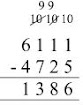So, a farm worker in Madhya Pradesh will get Rs 1,386 more than a farm worker in Orissa after working for 9 weeks.

Page No 176:

Question 1: Satish is a 13-year-old boy. His father had taken a loan for farming. But the crops failed. Now Satish's mother has to pay Rs 5000 every month for the loan. Satish started working --- he looked after 17 goats of the village. He earns Rupee 1 every day for one goat.

• How much will he earn in one month?
• Does he earn enough to help pay the loan every month?
• How much will he earn in one year?

• Earnings of Satish for looking after one goat for 1 day = Re I

It is given that Satish looks after 17 goats of the village in a day.

∴ Earnings of Satish for looking after 17 goats for 1 day = Re 1 × 17 = Rs 17 Number of days in 1 month = 30 Earnings of Satish for looking after 17 goats for 1 month = 30 x Rs 17 = Rs 510

Working:

30
X 17
210   (30×7)
+ 300   (30 × 10)
510

Thus, Satish will earn Rs 510 in one month.

• Satish's mother has to pay Rs 5,000 every month for the loan.

Thus, Satish does not earn enough to help pay the loan every month.

• Number of months in 1 year = 12

∴ Earnings of Satish for looking after 17 goats for 12 months = Rs 510 × 12 = Rs 6,120

Working:

510
x 12
1020  (510x 2)
+5100  (510 x 10)
6120

Thus, Satish will earn Rs 6,120 in one year for looking after 17 goats.

Question 2: To help farmers the State Government gave cows. Kamla Bai Gudhe also got a cow. The cost of the cow was Rs 17,500. She had to pay Rs 5,500 and the government spent the rest of the money. • How much did the government spend on the cow? • If 9 people from her village got cows, how much did the government spend in all? But Kamla Bai was not happy, she had to spend Rs 85 every day on the cow. She made some money by selling the milk. But still she wanted to sell the cow. • If Kamla Bai spends Rs 85 a day, find out how much she will spend in one month. • The cow gives 8 litre of milk every day. How much will it give in one month? • If the milk is sold at Rs 9 per litre, how much money will Kamla Bai make in one month?_____ So the money spent on keeping the cow was Rs _____ Money earned by selling the milk Rs_____Which is more ---- money spent on the cow or money earned form it? How much? Explain why she wanted to sell the cow.

• Cost of the cow = Rs 17,500 Money spent by Kamla Bai on the cow = Rs 5,500 It is given that the rest of the money was spent by the government. ∴ Money spent by the government on the cow = Rs 17500 − 5500 = Rs 12,000

Working:

17500
− 5500
12000

Thus, the government spent Rs 12,000 on one cow.

• Money spent by the government on I cow Rs 12,000 ∴ Money spent by the government on 9 cows = Rs 12000 × 9 = Rs 1,08,000

Working:

12000
×9
108000

Thus, the government spent Rs 1,08,000 on nine cows.

• Money spend by Kamla Bai on the cow in 1 day = Rs 85 Number of days in 1 month = 30. ∴Money spend by Kamla Bai on the cow in 30 days = Rs 85 x 30= Rs 2,550

Working:

85
× 30
00  (85 × 0)
+2550  (85 x 30)
2550

Thus, Kamla Bai will spend Rs 2,550 on the cow in one month.

• Amount of milk given by the cow in 1 day = 8 litre. Amount of milk given by the cow in 30 days = 30 x 8 litres = 240 litres

Working:

30
× 8
240

Thus, the cow will give 240 litres of milk in one month.

• Selling price of one litre of milk = Rs 9 We know that the cow gives 240 litres of milk in one month... Selling price of 240 litres of milk = 240 × Rs 9 = Rs 2,160

Working:

240
× 9
2160

Thus, Kamla Bai will earn Rs 2,160 by selling 240 litres of milk in one month.

Money spent on keeping the cow = Rs 2,550 Money earned by selling the milk = Rs 2,160 Thus, the money spent on keeping the cow is more than the money earned by selling the milk. Now, we will find the difference between the money spent on keeping the cow and the money earned by selling the milk.

254510  − 2160 = 0390

So, the money spent on keeping the cow is Rs 390 more than the money earned by selling the milk.

She wanted to sell the cow because she had to spend more money on the cow compared to her earnings by selling the milk.

Page No 177:

Question 1: (a) Sukhi works on a farm. He is paid Rs 98 for one day. If he works for 52 days, how much will he earn?

(b) Hariya took a loan to build his house. He has to pay back Rs 2,750 every month for two years. How much will he pay back in 2 years?

(c) Ratiram is milk seller in the city. He sells 13 litres of milk every day at Rs 23 per litre. How much does he earn?

(d) A farmer sells 1 litre of milk for Rs 11. In one month he sells 210 litres of milk. How much does he earn in a month?

(e) A company sells 1 litre of packed water for Rs 12. A shopkeeper buys 240 litres of packed water. How much does he pay?

(a) Money earned by Sukhi in 1 day = Rs 98. ∴ Money earned by Sukhi in 52 days = Rs 52 x 98 = Rs 5,096

Working:

52
X 98
416  (52 x 8)
+4680  (52 x 90)
5096

Thus, Sukhi will earn Rs 5,096 in 52 days.

(b) Money paid by Hariya in a month = Rs 2,750 It is given that Hariya has to pay Rs 2,750 every month for 2 years. Number of months in 1 year = 12 Number of months in 2 years = 2 x 12 = 24 Now, Money to be paid by Hariya in 24 months = 24 x Rs 2750 = Rs 66,000

Working:

2750
× 24
11000  (2750 × 4)
+55000  (2750 × 20)
66000

Thus, Hariya will pay Rs 66,000 in 2 years.

(c) Earnings of Ratiram by selling 1 litre of milk = Rs 23. ∴ Earnings of Ratiram by selling 13 litres of milk = Rs 23 × 13 = Rs 299

Working:

23
X13
69  (23 × 3)
+230  (23 × 10)
299

Thus, Ratiram earns Rs 299 after selling 13 litres of milk every day.

(d) Earnings of the farmer by selling 1 litre of milk = Rs 11 It is given that the farmer sells 210 litres of milk in 1 month. ∴ Earnings of the farmer in 1 month = 210 ×Rs 11 = Rs 2,310

Working:

210
X11
210  (210 x 1)
+2100  (210 x 10)
2310

Thus, the farmer earns Rs 2,310 in a month.

(e) Selling price of 1 litre of packed water = Rs 12 It is given that the shopkeeper buys 240 litres of packed water. ∴ Money paid by the shopkeeper = 240 Rs × 12 = Rs 2,880

Working:

240
X 12
480   (240 x 2)
+2400   (240 x 10)
2880

Thus, the shopkeeper pays Rs 2,880 for 240 litres of packed water.

Page No 178:

Question 1: Look for the pattern and take this forward.

(0x9) + 1=1

(1 × 9) + 2=11

(12 x 9) + 3= 111

(123 × 9) + 4 =

(1234 x 9) + 5 =

(12345 x 9) + 6 =

(0 × 9) + 1 = 1

(1 × 9) + 2 = 11

(12 x 9)+3=111

(123 x 9) + 4 = 1111

(1234 × 9) + 5 = 11111

(12345 x 9) + 6 = 111111

0 × 9 +1 =11 × 9 + 2 =1112 × 9 + 3 = 111123 × 9 + 4 =11111234 × 9 + 5 =1111112345

X 9 + 6 =111111

Question 2: Each letter a, b, c here stands for a number.

aaa
Xaaa
aaa
aaa0
+aaa00
abcba

Take a = 1, then find what the numbers b and c will be.

111
×111
111   (111 x 1)
1110  (111 x 10)
+11100  (111 x 100)
12321

Thus, we get b = 2, c=3

Question 3: Tricks with your age. Write your age ____ Multiply it by 7____ Again multiply the answer by 13____ Multiply again that answer by 11____ Now look at your last answer. Can you find your age in that answer? How many times does your age show in the answer? Now try this trick with other people.

My age is 10 years. Product of my age and 7 = 10 x 7 = 70 Product of 70 and 13 = 70 × 13 = 910

Working:

70
X 13
210  (70×3)
+700  (70 x 10)
910

Product of 910 and 11 = 910 x 11= 10,010

910
x 11
910  (910×1)
+9100  (910 x 10)
10010

Yes, I can find my age in the final answer. My age appears two times in the answer.

Page No 179:

Question 1: Going round and round!

142857 × 1

142857 × 2

142857 × 3

142857 × 4

142857 × 5

Do you find a pattern in all these answers? Discuss this with your friends.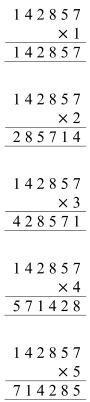No, we cannot find a pattern in the answer.

Disclaimer: The discussion may vary from student to student, based on his/her experience.

Question 2: Dolma took a loan from a friend to buy a moped for Rs 9,588. She has to pay it back in equal amounts every month for six months.How much will she have to pay every month? She asked her children to calculate.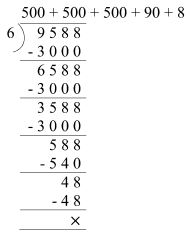Her son started this way. Now you complete it. 1000  + 500  + 90  + 8 Will both of them get the same answer? Discuss.

The method followed by Dolma's son is given below.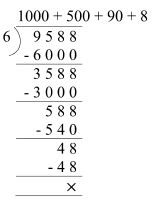Answer calculated by her daughter = 500 + 500 + 500 + 90 + 8 = 1598 Answer calculated by her son = 1000 + 500 + 90 + 8 =1598 So, both the children get the same answer. Thus, Dolma has to pay Rs 1,598 every month for 6 months to her friend.

Page No 180:

Question 1: Try to solve these using as few steps as you can. (a) 4228 ÷ 4 (b) 770 ÷ 22 (c) 9872 ÷ 8 (d) 672 ÷ 21 (e) 772 ÷ 7 (f) 639 ÷ 13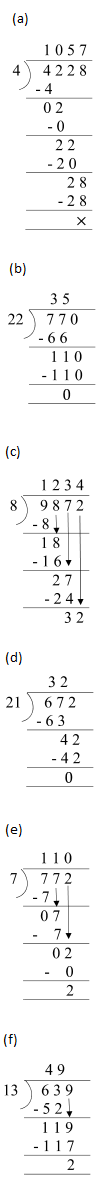Page No 181:

Question 1: Isha has Rs 1000 with her. She wants to buy petrol. One litre of petrol costs Rs 47. How many litres can she buy? Money with Isha = Rs 1000 Cost of 1 litre = Rs 47 Litres of petrol she can buy = Rs 1000 ÷ Rs 47? Isha can buy____ litres of petrol. Find out If Isha comes to your city, how much petrol can she buy with the same money?

Money with Isha = Rs 1,000 Cost of 1 litre of petrol = Rs 47 Number of litres of petrol Isha can buy = 1000 ÷ 47

Working: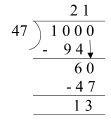Quotient = 21 Remainder = 13 Thus, Isha can buy 21 litres of petrol with Rs 1,000.

Cost of 1 litre of petrol in my city = Rs 50 Number of litres of petrol Isha can buy from Rs 1,000 = 1000 ÷ 50=20

Working: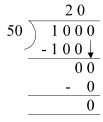Thus, Isha can buy 20 litres of petrol in my city.

Disclaimer: The price of petrol may vary from place to place. The answer provided here is for reference only.

Question 2: Children are happy today. They are celebrating Children's Day. Each child will be given 4 coloured pencils from school. The school has got 969 pencils. To find out how many children can get pencils the teacher asks them to divide.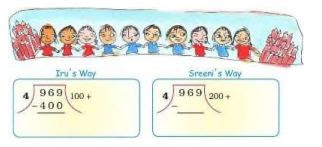Complete Iru's and Sreeni's way of division. What is the answer you get?

Iru's way: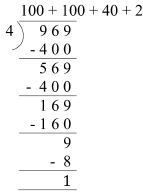Sreeni's way:Page No 183:

Question 1:

• 576 books are to be packed in boxes. If one box has 24 books, how many boxes are needed? 836 people are watching a movie in a hall. If the hall has 44 rows, how many people can sit in 1 row? A gardener bought 458 apple trees. He wants to plant 15 trees in each row. How many rows can he plant? How many trees would be left over?

• Number of boxes required to pack 24 books = 1

There are 576 books that need to be packed in boxes. ∴ Number of boxes required to pack 576 books = 576 ÷ 24= 24

Working: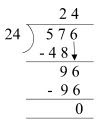Thus, 24 boxes are required to pack 576 books.
• Total number of people watching movie in the hall = 836

Number of rows in the hall = 44. ∴ Number of people sitting in 1 row = 836 ÷ 44 = 19

Working: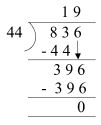Thus, 19 people are sitting in 1 row of the hall.
• Total number of apple trees bought by the gardener = 458 Now, the gardener wants to plant 15 trees in each row.

∴ Number of rows of apple trees that can be planted by the gardener = 458 ÷ 15

Working: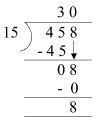Quotient = 30 Remainder = 8 So, the gardener can plant 30 rows of apple trees and 8 trees will be left.

Question 2: Shyamli bought a battery. She read on it 'Life: 2000 hours'. She uses it throughout the day and the night. How many days will the battery run?

Life of the battery = 2000 h Number of hours in 1 day = 24 Number of days for which the battery can run = 2000 ÷ 24 = 83

Working: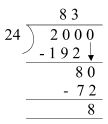Quotient = 83 Remainder = 8 So, the battery can run for 83 days.

Question 3: A tank is full of 300 L of water. How much water will be filled in 25 tanks? If 15 buckets can be filled with one tank of water, how many buckets in all can be filled with the water in 25 tanks?

Amount of water in 1 tank = 300 L

∴ Amount of water in 25 tanks = 25 × 300 = 7500 L

Number of buckets that can be filled with I tank of water = 15

∴ Number of buckets that can be filled with 25 tanks if water = 25 × 15 = 375

Working: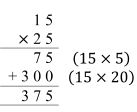Thus, 375 buckets can be filled with 25 tanks of water.

Question 4: There are 28 laddoos in 1 kg. How many laddoos will be there in 12 kg? If 16 laddoos can be packed in 1 box, how many boxes are needed to pack all these laddoos?

Number of laddoos in 1 kg = 28. ∴ Number of laddoos in 12 kg = 12 × 28 = 336

Working:

12
× 28
96  (12×8)
+240  (12 x 20)
336

Thus, there are 336 laddoos in 12 kg.

Number of boxes needed to pack 16 laddoos = 1 ∴ Number of boxes needed to pack 336 laddoos = 336 ÷16=21

Working: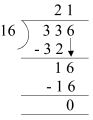Thus, 21 boxes are needed to pack all the laddoos.

Question 5: There are 26 rooms in a school. Each room has 4 plants. If each plant needs 2 cups of water, how much water do we need for all the plants?

Total number of rooms in the school = 26 Number of plants in each room = 4 Total number of plants in the school = 4 × 26 = 104 Working:

26 × 4 = 104 Number of cups of water required by 1 plant = 2 Number of cups of water required by 104 plants = 2 × 104 = 208

Working:

104
× 2
208

Thus, we need 208 cups of water for all the plants.

Page No 184:

Question 1: Each line gives a story. You have to choose the question which makes the best story problem. (1) A shopkeeper has 50 boxes. There are 48 fruits in one box.

(2) 352 children from a school went on a camping trip. Each tent had a group of 4 children.

(a) How many children did each tent have?

(b) How many tents do they need?

(c) How many children in all are in the school?

(3) A shopkeeper has 204 eggs. He puts them in egg trays. Each tray has 12 eggs.

(a) How many more eggs will he need?

(b) How many fresh eggs does he sell?

(c) How many egg trays does he need?

(4) The cost of one book is Rs 47. Sonu buys 23 books.

(a) How much money does she have?

(b) How much money does she pay for the books?

(c) What is the cost of 47 books?

1.(a) How many children did each tent have?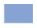(b) How many tents do they need?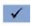2.(b) How many children in all are in the school?(a) How many more eggs will he need?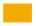(b) How many fresh eggs does he sell?3.(b) How many egg trays does he need?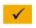(a) How much money does she have?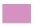(b) How much money does she pay for the books?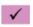4.(b) What is the cost of 47 books?Page No 186:

Question 1: Do these divisions. Check your results by multiplication. (a) 438 ÷ 9 (b) 3480 ÷ 12 (c) 450 ÷ 7 (d) 900 ÷ 10 (e) 678 ÷ 6 (f) 2475 ÷ 11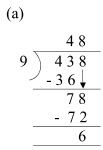Divisor = 9 Quotient = 48 Remainder = 6 Checking: Divisor × Quotient

+ Remainder 9 × 48 + 6 = 432 + 6= 438 = Dividend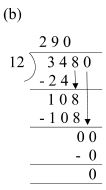Divisor = 12 Quotient = 290 Remainder = 0 Checking: Divisor × Quotient + Remainder 12 × 290 + 0=3480 + 0=3480 = Dividend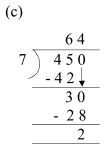Divisor = 7 Quotient = 64 Remainder = 2 Checking: Divisor × Quotient + Remainder = 7 × 64 + 2 = 448+2=450 = Dividend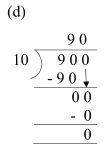Divisor = 10 Quotient = 90 Remainder = 0 Checking: Divisor × Quotient + Remainder 10 × 90 + 0 = 900 + 0 = 900 = Dividend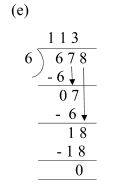Divisor = 6 Quotient = 113 Remainder = 0 Checking: Divisor × Quotient + Remainder 6 × 113 + 0 = 678 + 0 = 678 = Dividend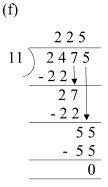Divisor = 11 Quotient = 225 Remainder = 0 Checking: Divisor × Quotient + Remainder 11 × 225 + 0 = 2475 + 0 = 2475 = Dividend

Question 2: Solve the given sums and colour the answers in the grid given below See what you find.

21 × 16        15×7            93 × 2           17×5           10 × 10

26 × 26       77 × 10        50 × 10         11 × 11        59 × 7        31 × 19

85 × 30       64 × 42       3200 ÷ 40      19 × 3          248 ÷ 8

432 ÷ 18    729 ÷ 9      825 ÷ 5        221 ÷ 13      576 ÷ 12

288 ÷ 4      869 ÷ 11    847 ÷ 7        981 ÷ 3          475 19